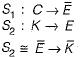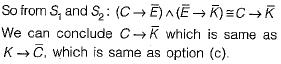Courses

# Mathematical Logic (Advance Level) - 1

## 13 Questions MCQ Test Mock Test Series for Electrical Engineering (EE) GATE 2020 | Mathematical Logic (Advance Level) - 1

Description
This mock test of Mathematical Logic (Advance Level) - 1 for GATE helps you for every GATE entrance exam. This contains 13 Multiple Choice Questions for GATE Mathematical Logic (Advance Level) - 1 (mcq) to study with solutions a complete question bank. The solved questions answers in this Mathematical Logic (Advance Level) - 1 quiz give you a good mix of easy questions and tough questions. GATE students definitely take this Mathematical Logic (Advance Level) - 1 exercise for a better result in the exam. You can find other Mathematical Logic (Advance Level) - 1 extra questions, long questions & short questions for GATE on EduRev as well by searching above.
QUESTION: 1

### Which one of the following is false? Read ∧ as AND, v as OR, ~ as NOT, → as one way implication and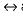as two way implication.

Solution: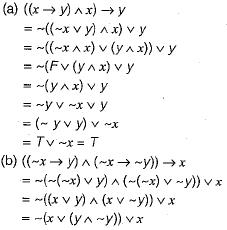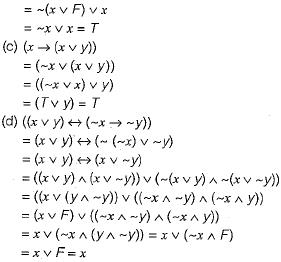QUESTION: 2

### What is the converse of the following assertion? I stay only if you go

Solution:

Let p : l stay
q ; you go
I stay only if you go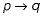Converse of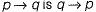Now convert the answers one-by-one into boolean form. Only option (a) i.e. “1 stay if you go” converts to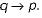QUESTION: 3

### Consider two well-formed formulas in propositional logic: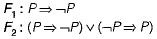Which of the following statements is correct?

Solution: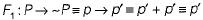So F1 is contingency. Hence, F1 is satisfiable but not valid.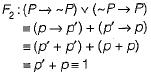So F2 is tautology and therefore valid.

QUESTION: 4

“If X then Y unless Z” is represented by which of the following formulas in propostional logic? ("_") is negation, “∧” is conjunction, and "→" is implication)

Solution:

If X then Y unless Z is represented by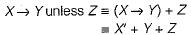Now convert the answers one-by-one into boolean form only choice (a) converts to X'+ Y + Z as can be seen below: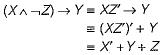QUESTION: 5

Let P, Q and, R be three atomic propositional assertions. Let X denote ( P v Q ) → R and Y denote (P → R) v (Q → R). Which one of the following is a tautology?

Solution: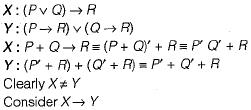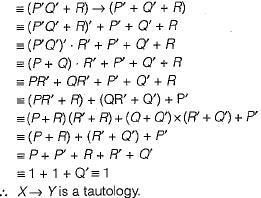QUESTION: 6

Which one of the following is the most appropriate logical formula to represent the statement:
“Gold and silver ornaments are precious”
The following notations are used:
G(x): x is a gold ornament
S(x): x is a silver ornament
P(x): x is precious

Solution:

The correct translation of "Gold and silver ornaments are precious ” is choice (d)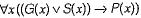which is read as “if an ornament is gold or silver, then it is precious”.
Now since a given ornament cannot be both gold and silver at the same time.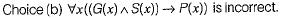QUESTION: 7

Suppose the predicate P(x, y, t) is used to represent the statement that person x can fool person y at time t. Which one of the statements below expresses best the meaning of the formula​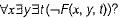Solution: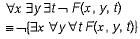≡  it is not true that (someone can fool all people at all time)
≡  no one can fool everyone all the time

QUESTION: 8

What is the logical translation of the following statements?
“None of my friends are perfect"

Solution:

None of my friends are perfect i.e., all of my friends are not perfect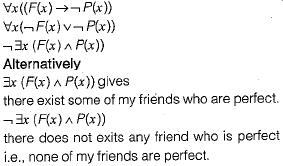QUESTION: 9

Consider the statement:
"Not all that glitters is gold"
Predicate glitters (x) is true if x glitters and predicate gold (x) is true if x is gold. Which one of the following logical formulae represents the above statement?

Solution:There exist gold which is not glitter i.e. not all golds are glitters.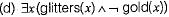Not all that glitters is gold i.e., there exist some which glitters and which is not gold.

QUESTION: 10

Which one of the following propositional logic formulas is TRUE when exactly two of p, q, and r are TRUE?

Solution: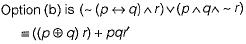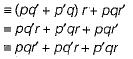This is exactly the min-term form of a logical formula which is true when exactly two variables are true (only p, q true or only p, r true or only q, r true).

QUESTION: 11

Which one of the following Boolean expressions is NOT a tautology?

Solution: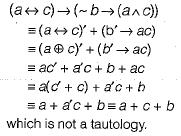QUESTION: 12

Consider the following statements:
P : Good mobile phones are not cheap
Q : Cheap mobile phones are not good
L : P implies Q
M : Q implies P
N : P is equivalent to Q
Which one of the following about L, M and N is CORRECT?

Solution:

g : mobile is good
c : mobile is cheap
P : Good mobile .phones are not cheap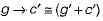Q : Cheap mobile phones are not good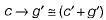∴ Both P and Q are equivalent.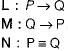Since both P and Q are equivalent, all three of L, M, N are true.

QUESTION: 13

Consider the following two statements:
S1: If a candidate is known to be corrupt, then he will not be elected.
S2: If a candidate is kind, he will be elected.
Which one of the following statements follows from S1 and S2 as per sound inference rules of logic?

Solution:

C : Person is corrupt
K : Person is kind.
E : Person is elected